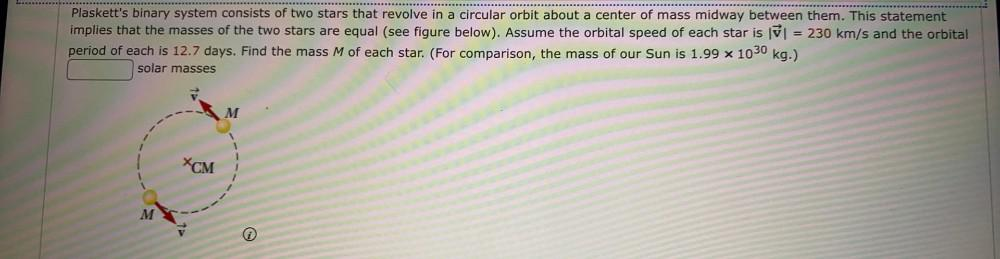Question:

# Plaskett's binary system consists of two stars that revolve in a circular orbit about a center of mass midway between them. ThisPlaskett's binary system consists of two stars that revolve in a circular orbit about a center of mass midway between them. This statement implies that the masses of the two stars are equal (see figure below). Assume the orbital speed of each star is = 230 km/s and the orbital period of each is 12.7 days. Find the mass M of each star. (For comparison, the mass of our Sun is 1.99 % 1030 kg.) solar masses M XCM M